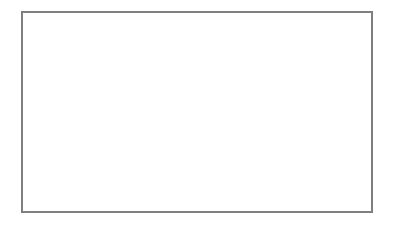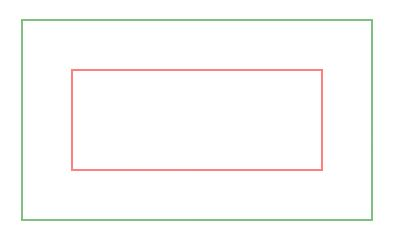# HTML | canvas strokeRect() Method

• Last Updated : 29 Apr, 2019

The strokeRect() method is used to draw the rectangle in a given color. The default color of the stroke is black.

Syntax:

Attention reader! Don’t stop learning now. Get hold of all the important HTML concepts with the Web Design for Beginners | HTML  course.

`context.strokeRect(x, y, width, height)`

Parameters:

• x: It stores the x-coordinate of top-left corner of rectangle.
• y: It stores the y-coordinate of top-left corner of rectangle.
• width: It stores the width in pixel.
• height: It stores the height in pixel.

Example-1:

 ```<``html``>`` ` `<``head``>``    ``<``title``>``        ``HTML canvas strokeRect() Method``    `````` ` `<``body``>``    ``<``canvas` `id``=``"GFG"``            ``width``=``"500"` `            ``height``=``"300"``>``  ```` ` `    ``<``script``>``        ``var x =``            ``document.getElementById("GFG");``        ``var contex = x.getContext("2d");``        ``contex.strokeRect(50, 50, 350, 200);``        ``contex.stroke();``    ```` ` ``` ` ``

Output:Example-2:

 ```<``html``>`` ` `<``head``>``    ``<``title``>``        ``HTML canvas ``      ``strokeRect() Method``    `````` ` `<``body``>``    ``<``canvas` `id``=``"GFG"` `            ``width``=``"500"``            ``height``=``"300"``>``  ```` ` `    ``<``script``>``        ``var x =``            ``document.getElementById("GFG");``        ``var contex =``            ``x.getContext("2d");``        ``contex.strokeStyle = "green";``        ``contex.strokeRect(50, 50, 350, 200);`` ` `        ``contex.strokeStyle = "red";``        ``contex.strokeRect(100, 100, 250, 100);``        ``contex.stroke();``    ```` ` ``` ` ``

Output:Supported Browsers: